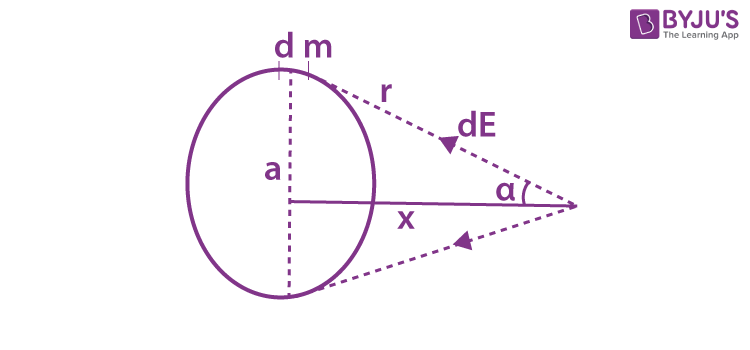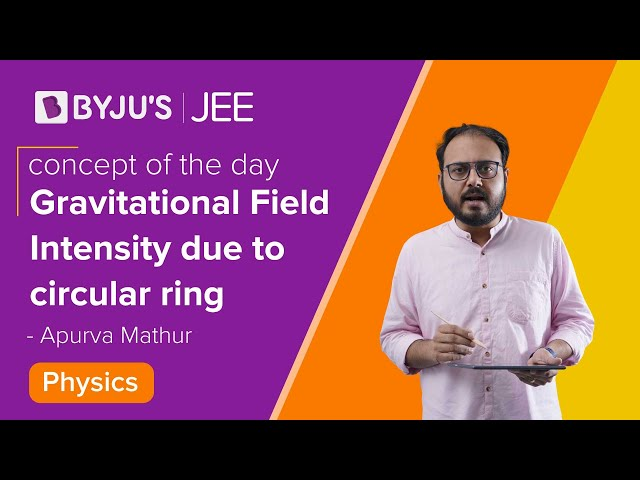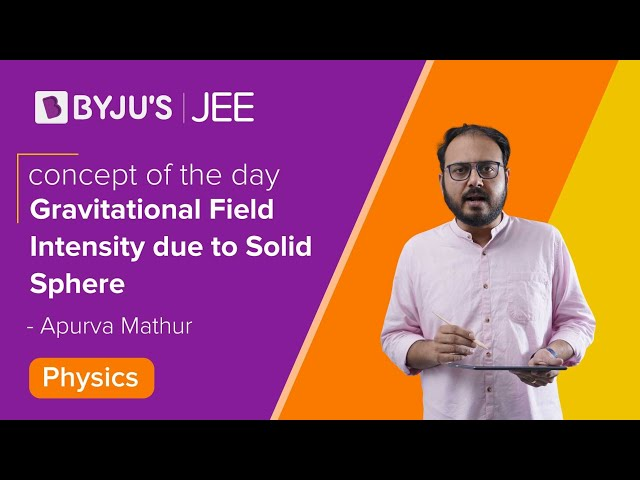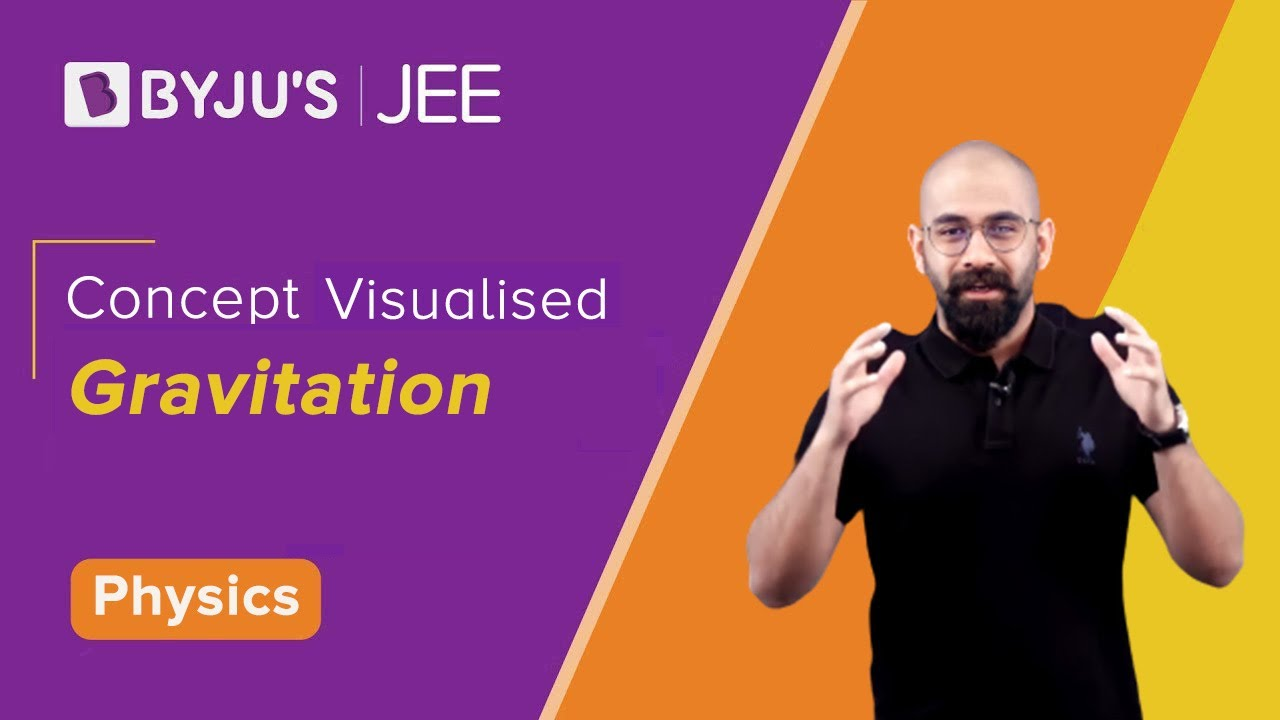Win up to 100% scholarship on Aakash BYJU'S JEE/NEET courses with ABNAT Win up to 100% scholarship on Aakash BYJU'S JEE/NEET courses with ABNAT

# Gravitational Field Intensity - Point Mass, Ring, Spherical Shell, Solid Sphere

## What Is Gravitational Field?

For a non-contact force, the source mass and test mass interact with each other by means of a gravitational field. You can think of gravitational force as a “command” and gravitational field like the dialogue or speech used in giving the command.

## What Is Gravitational Field Intensity?

The strength of the gravitational field is known as gravitational field intensity. It is the gravitational force acting on a unit test mass.

Eg = F/m

Or

$$\begin{array}{l}E_g = \left[-\frac{\frac{GMm}{r^2}}{m}\right] \hat{r}\end{array}$$

⇒ Gravitational Field Intensity

$$\begin{array}{l}\mathbf{E_g = \left[-\frac{GM}{r^2}\right]\hat{r}}\end{array}$$

Where

$$\begin{array}{l}\hat{r}\ \text{represents the unit vector along the radial direction,}\end{array}$$

$$\begin{array}{l}\vec{r}=x\hat{i}+y\hat{j}+z\hat{k}\end{array}$$
represents the position vector of the test mass from the source mass.

The gravitational field intensity depends only upon the source mass and the distance of the unit test mass from the source mass.

• The unit of gravitational field intensity is N/kg.
• The dimensional formula is given by [M0L1T-2].

The dimensional formula of gravitational field intensity is identical to the acceleration (preferably, we call it as acceleration due to gravity from the view of gravitation).

The superposition principle extends to gravitational field intensities as,

E = E1 + E2 + E3 + . . . . . . . + En

Where, E1, E2, E3, . . . . . En are the gravitational field intensities at a point due to n particles in a system.

In a system, the mass is always distributed in two different ways:

• Discrete mass distribution
• Continuous mass distribution

For a discrete mass distribution:

$$\begin{array}{l}\vec{E} = \sum_{i = 1}^{n}E_{i}\end{array}$$

For a continuous mass distribution:

$$\begin{array}{l}\vec{E} = \int_{i}^{f} dE\end{array}$$

dE is the gravitational field intensity due to an elementary mass dm. The gravitational field formula is expressed as,

g = F/m

Where,

F = gravitational force, and m = mass of the object.

### Gravitational Field Intensity of a Point Mass

Consider a point mass M, the gravitational intensity at a distance ‘r’ from it is given by

$$\begin{array}{l}E_g = \left[-\frac{GM}{r^2}\right]\hat{r}\end{array}$$

### Gravitational Field Intensity due to Ring

Let us consider a ring of mass M, having radius ‘a’; the gravitational field at a distance x along its axis is found as follows:Consider a small length element along the circumferential length of the ring which has a mass ‘dm’; the field intensity due to this length element is given by,

dE = Gdm/r2

The vertical components of the fields cancel each other due to the symmetry of the ring, and only horizontal components survive and add up to,

$$\begin{array}{l}E = \int_{0}^{2\pi}\frac{Gdm}{r^{2}}cos\alpha\end{array}$$

Since

$$\begin{array}{l}cos\alpha =\frac{x}{\sqrt{{{x}^{2}}+{{a}^{2}}}}\end{array}$$
,

On substituting, we get

$$\begin{array}{l}E=\frac{GMx}{{{\left( {{x}^{2}}+{{a}^{2}} \right)}^{{}^{3}/{}_{2}}}}\end{array}$$

#### Gravitational Field Intensity due to Circular Ring – Video Lesson## Gravitational Field due to Uniform Spherical Shell

Consider a thin uniform spherical shell of radius ‘R’, and mass ‘M’ situated in a space. A 3D object divides space into 3 parts:

• Inside the spherical shell.
• On the surface of the spherical shell.
• Outside the spherical shell.

Our problem is to find out the value of gravitational field intensity in all these 3 regions.

### Outside the Spherical Shell

Consider a unit test mass at a point ‘P’, which is at a distance ‘r’ from the centre of the spherical shell. Draw an imaginary spherical shell such that point ‘P’ lies on the surface of it.

As we know, the gravitational field intensity at a point depends only upon the source mass and the distance of the point from the source mass. We can say that within the imaginary sphere, the source mass is M, and the distance of separation is ‘r’. From this, we get

E = -GM/r2

⇒ E ∝ -1/r2

### On the Surface of Spherical Shell

Consider a unit test mass at a point ‘P’, which lies on the surface of the spherical shell at a distance ‘r’ from the centre of the spherical shell, then r = R. As discussed above, the gravitational field intensity on the surface of the spherical shell is given by,

E = -GM/R2

⇒ E = Constant

⇒ Check: Acceleration Due to Gravity

### Inside the Spherical Shell

If we consider a point inside the spherical shell, the entire mass of the shell lies above the point. Draw an imaginary spherical shell about point ‘P’; the source mass within this imaginary sphere will be zero.

We know that if the source mass is equal to zero, the gravitational field intensity is also equal to zero.

∴ E = 0

Conclusions:

 Position of Point ‘P’ Gravitational Field Intensity Inside the spherical shell (r < R) E = 0 On the surface of the spherical shell (r = R) E = -GM/R2 Outside the spherical shell ( r > R) E = -GM/r2

## Gravitational Field due to Uniform Solid Sphere

Consider a uniform solid sphere of radius ‘R’ and mass ‘M’. Let us find out the value of gravitational field intensity in all these 3 regions:

• Inside the solid sphere.
• On the surface of a solid sphere.
• Outside the solid sphere.

### Outside the Solid Sphere

To find the gravitational field intensity at a point ‘P’, which is at a distance ‘r’ from the centre of outside the solid sphere, consider an imaginary sphere about ‘P’, which encloses the entire mass ‘M’.

∴ E = – GM/r2

⇒ E ∝ -1/r2

### On the Surface of a Solid Sphere

To find the gravitational field intensity at a point ‘P’ situated on the surface of the solid sphere,

Distance to the point on the surface is r = R.

Then, E = -GM/R⇒ E = Constant.

### Inside the Solid Sphere

To find the gravitational influence at a point ‘P’ situated inside the uniform solid sphere at a distance ‘r’ from the centre of the sphere. If we draw an imaginary sphere about this point, the mass present within this imaginary sphere is given by ‘m’.

For a volume of (4/3) πR3, the mass present is M; for a volume of (4/3) πr3, the mass present is ‘m’.

As the density of the solid sphere remains constant throughout,

m = M × (r3/R3)

Then, the gravitational field intensity at point ‘P’ inside the solid sphere at a distance ‘r’ from the centre of the sphere is given by,

E = -Gm/r2

Where m is the source mass present within the imaginary sphere drawn about point ‘P’. By substituting the value of m in the above equation, we get

E = -GMr/R3

⇒ E ∝ -r

Conclusions:

 The Position of Point ‘P’ Gravitational Field Intensity Inside the uniform solid sphere (rR) E = -GM/r2

#### Gravitational Field Intensity due to Solid Sphere – Video Lesson## Solved Examples

Example 1: If the gravitational force and mass of a substance are 10 N and 5 kg, determine the gravitational field.

Solution:

The given parameters are, F = 10 N and m = 5 kg

The formula for gravitational field intensity is given by,

g = F/m = 10/5 = 2 N/kg

Example 2: Calculate the gravitational field if the mass and force of a substance are given as 6 kg and 36 N, respectively.

Solution:

The given parameters are, F = 36 N and m = 6 kg

The formula for gravitational field intensity is expressed by, g = F/m = 36/6 = 6 N/kg.

## Gravitation## Gravitational Potential Energy## Frequently Asked Questions on Gravitational Field Intensity

Q1

N/kg

Q2

### What is a gravitational field?

The space or region around a body within which other bodies experience its gravitational force of attraction is called a gravitational field.

Q3

### Define gravitational field intensity.

The intensity or strength of the gravitational field is defined as the force experienced by a body of unit mass placed at that point.

Q4

### What is meant by gravitational potential?

Gravitational potential at a point in the gravitational field of a body is defined as the amount of work done in bringing a body of unit mass from infinity to that point in the gravitational field.

Test Your Knowledge On Gravitational Field Intensity!